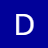### 变量的引用

PHP的引用允许你用两个变量来指向同一个内容。

```<?
\$a="ABC";
\$b =&\$a;
echo \$a;//这里输出:ABC
echo \$b;//这里输出:ABC
\$b="EFG";
echo \$a;//这里\$a的值变为EFG 所以输出EFG
echo \$b;//这里输出EFG
?>```

### 函数的传址调用

```functiontest(&\$a){
\$a=\$a+100;
}
\$b=1;
echo\$b;//输出1
test(\$b);//这里\$b传递给函数的其实是\$b的变量内容所处的内存地址，通过在函数里改变\$a的值就可以改变\$b的值了
echo "<br />";
echo\$b;//输出101```

### 函数的引用返回

```function&test(){
static\$b=0;//申明一个静态变量
\$b=\$b+1;
echo\$b;
return\$b;
}
\$a=test();//这条语句会输出\$b的值为1
\$a=5;
\$a=test();//这条语句会输出\$b的值为2
\$a=&test();//这条语句会输出\$b的值为3
\$a=5;
\$a=test();//这条语句会输出\$b的值为6```

\$a=test()方式调用函数，只是将函数的值赋给\$a而已，而\$a做任何改变都不会影响到函数中的\$b。而通过\$a=&test()方式调用函数呢，他的作用是将return\$b中的\$b变量的内存地址与\$a变量的内存地址指向了同一个地方即产生了相当于这样的效果(\$a=&b;)所以改变\$a的值也同时改变了\$b的值，所以在执行了

```\$a=&test();
\$a=5;```

### 对象的引用

```<?
class a{
var \$abc="ABC";
}
\$b=new a;
\$c=\$b;
echo \$b->abc;//这里输出ABC
echo \$c->abc;//这里输出ABC
\$b->abc="DEF";
echo \$c->abc;//这里输出DEF
?>```

```<?php
\$a = 1;
\$b =& \$a;
unset (\$a);
?>```

```<?php
\$var =& \$GLOBALS["var"];
?>```

\$this在一个对象的方法中，\$this永远是调用它的对象的引用。

php中对于地址的指向(类似指针)功能不是由用户自己来实现的，是由Zend核心实现的，php中引用采用的是"写时拷贝"的原理，就是除非发生写操作，指向同一个地址的变量或者对象是不会被拷贝的。

```\$a="ABC";
\$b=\$a;```

`\$a="EFG";`

×

×

#### 评论列表 :共有1人吐槽, 3184人围观